### Home > CALC > Chapter 6 > Lesson 6.4.1 > Problem6-132

6-132.
1. For each graph below: Homework Help ✎

1. Trace f(x) on your paper and write a slope statement for f(x).

2. Sketch the graph of f ′(x) using a different color.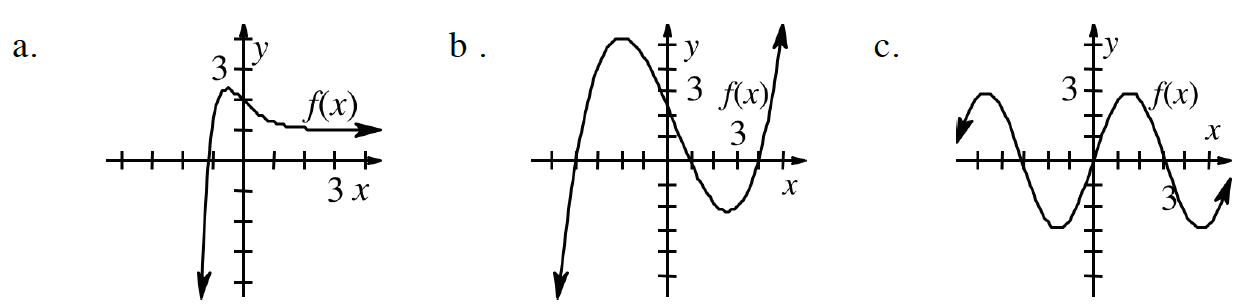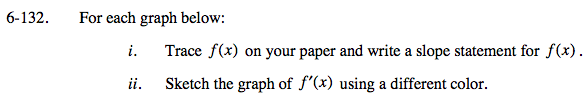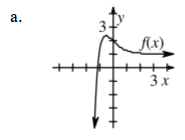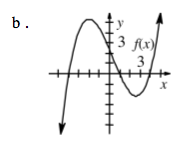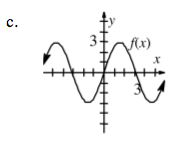Slope statements should tell a story, starting at the left side of the graph and ending at the right side.
There should be a description of where the function is increasing or decreasing and how the rate in which it is increasing or decreasing is changing.

Steep positive slopes on f(x) should have very high y-values on the graph of f '(x). While steep negative slopes on f(x) should have very low y-values on the graph of f '(x). Maxima and minima on f(x) have 0 slope, so they are roots on the graph of f '(x).